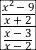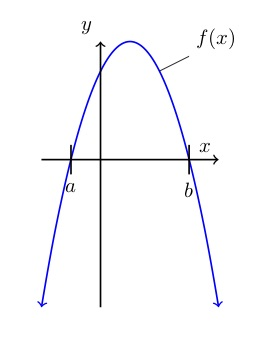## Question

Does the sequence converge?

## Solution

A sequence converges if there is a limit for its terms as the number terms goes to infinity.

Is there a limit of as goes to infinity? Let’s see:

. Since the limit as goes to infinity of equals 0, the limit as goes to infinity of .

There is a limit:. Therefore the sequence converges.

### CautionGraph of the 3n/(2n-1). Dots represent sequence values. Value approaches 3/2 as n gets large.

There may be confusion between convergence of a sequence and conversion of a series. Note the distinction: A sequence is a list of numbers: one number, then another number, then another, etc.; while a series is a sum of numbers: one number plus another number, plus another, etc. More confusing, there is a whole topic about convergence of series, with several kinds of tests for convergence.

To determine whether a sequence converges, don’t use the convergence tests that you use for series; instead, look at the limit. While a series converges only if the sum of the terms is limited, a sequence converges if its th term is limited as goes to infinity.

### Update

Paul Headley, who teaches at Northern Virginia Community College, has found an easier way to find the limit: L’Hopital’s rule. L’Hopital’s rule says that if the limits of both the numerator and the denominator of a rational function go to either zero or infinity, then the limit of the rational function is equal to the quotient of the limits of the derivatives of the numerator and the denominator.

In our example: To find the limit as goes to infinity of , start by noting that as goes to infinity, 3n and 2n-1 both go to infinity, so L’Hopital’s rule applies.

Next, replace the numerator and the denominator with their first derivatives, and you get . There’s the limit. Same answer, less sweat.

# System of Nonlinear Equations

## Question

Find the value or values of that are solutions to this system of equations:

## Solution

If the equations look familiar enough so that you can sketch them by hand, then doing so may help you get your arms around the problem. (The calculator provided for the exam does not have graphing capability, so if you want to see a graph you pretty much need to make it yourself. Learn more about it here.)A very rough sketch will do. I think my sketch (left) is pretty crude. For this problem, the first equation is for a circle with center at the origin and radius equal to the square root of 5. The square root of 5 is about 2.2, so the circle goes through points (2.2, 0); (0, 2); (-2.2, 0); and (0, -2.2), approximately.

The second is for a line with a slope of -1 and intercepts at   and . The solutions to the system lie at the points of intersection of the graphs, one in the second quadrant (where is negative and is positive) and the other in the fourth quadrant (where is positive and is negative).

The sketch is just to help you get your arms around the problem. If it doesn’t work for you, you can skip it — it’s not essential.

You can solve using substitution. If  then ; substitute  for  in the equation

 Simplify: Multiply out, subtract 5 from each side, and collect like terms. Divide both sides by 2. Factor Set each factor equal to 0 and solve.

That’s all you need to answer the question.

To check your answers, use the equation  to find the y-values that correspond to the x-values you found, and then substitute x- and y-values into the equation

This question is about nonlinear systems of equations. (The whole system gets labeled nonlinear even though one of the equations is linear.)

# How do you simplify a rational expression within a rational expression?

## Question

How do you simplify ?

## Solution

This is a complex fraction, a fraction with fractions in it. To be simplified it needs to have just one numerator and just one denominator.Treat this like the division problem it is. For fractions, division works like this: . . In other words, flip the bottom fraction and multiply. Factor where possible so you can see any places where cancellation is possible. The x – 3 term shows up in both numerator and denominator. Cancel it.

Are we finished? Maybe. That business in the question about being defined? The expression – either the original or the simplified version – is undefined for any value of xthat would make any of the three denominators in the original expression –  ,  , and  — equal to zero. The words “for which it is defined” just mean you can assume you’re not dealing with any of those values of x.

This question is about rational expressions, complex fractions, and domain constraints.

# What’s up (more), the parabola or the line?

## QuestionThe graph to the left shows the function f(x), a parabola – that is, a quadratic function. Not shown on the graph is function  in which c is a negative constant.

One statement below is true. Which one?

## Solution

What’s going on?  is the equation of a straight line. The line will cross the parabola in a couple of places and those intersections will determine which function is higher in various places. But all you know about function is that  and that c is negative.

Think of a line’s basic equation:  where b is the line’s y-intercept – that is, y’s value where . In this case, because  nothing, the y-intercept is 0. That means the line goes through the origin.

Also, you’re told that c is negative. c is in the role of m in the equation that is, it’s the slope. The negative slope means this line goes down as it goes to the right.

How steep is the line? You are given no information about that. Take a guess.

The brown line in the graph to the right shows what function g may look like. It may be steeper or less steep than shown,but it will cross the x-axis at the origin, and it will go down as it goes to the right, as shown.

Let’s go through all the possible answers to find the one true statement.

1. . At , as you can see from the graph, the parabola is above the x-axis, which means f(0) is a positive value. The line goes right through the origin – so . Thus which means it cannot be true that .
2. . At , function f crosses the x-axis. So . At , function g crosses the x-axis. So . That means f(a) is equal to g(0), so it’s not possible that f(a) is less than g(0).
3. . What’s happening at ? Function f crosses the x-axis there, so ; and function g has a positive value. That means g is more than f, so it must be that . Looks like that’s the answer, but let’s still check the others.
4. . We have established that . And since g(x) goes through the origin and slopes down, g must be less than zero at any x-value that is greater than 0 – like, for example, b. That means g is less than f, so it is not possible that f is less than g.
5. . As you can see from the graph, . And, as we said in response to answer #4, g(b) is less than 0. So f(b) must be more than g(b) – and it’s not possible that .